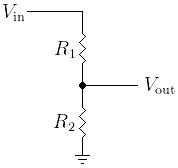# Gzalo.com

## Resistive divider calculator

Given the two values of the resistors and an input voltage, this page calculates the output voltage of the resistor divider.

R1: Ohms

R2: Ohms

Vin [Volts]

VoutA resistive divider (also called voltage divider) is a linear circuit that outputs a voltage (Vout) which is a fraction of the input voltage (Vin).

The circuit itself is based on two resistors in series, taking the middle node as the output. It's usually done to create a reference, or to attenuate a signal

Whenever a load is connected to the output node, a loading effect is produced. This means that the output voltage drops. To avoid this, the current flowing by the resistors should be higher than the one flowing to the load. The disadvantage of doing this is that efficiency is low, as most of that current disipates as heat.

The output resistance is the equivalent of the paralell of both resistor, Rout = (R1*R2)/(R1+R2).